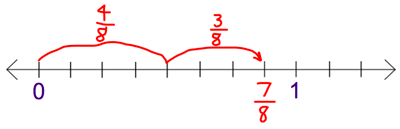# Number lines

This activity uses eighths, but can be adjusted to use other fractions and diagrams. Examples of same denominator problems are available.

Together the class constructs a number line from 0 to 2, labelled with eighths. The line should be labelled with both improper fractions and mixed numbers.

Present a contextual problem.

The family bought some pizzas. I ate $$\frac{4}{8}$$ of the pepperoni pizza and $$\frac{3}{8}$$ of the ham and pineapple pizza. How much pizza did I eat?

• Ask students to predict whether the answer will be less than, equal to, or more than one, and explain their reasons.
• Use questioning to scaffold the modelling of the problem with circles partitioned into eighths to represent the pizzas.
• On the number line, mark the two jumps to $$\frac{7}{8}$$. Display the equation $$\frac{4}{8}$$ + $$\frac{3}{8}$$ = $$\frac{7}{8}$$.Student work sample showing addition of fractions with related denominators.

Extend the problem.

Later I ate $$\frac{2}{8}$$ of the vegetarian pizza. How much pizza had I eaten altogether?

• Ask students to predict the answer, then model with the circle diagrams and the extra jump on the number line. Discuss the equivalence of $$\frac{9}{8}$$ and 1$$\frac{1}{8}$$.
• Display the equation: $$\frac{4}{8}$$ + $$\frac{3}{8}$$ + $$\frac{2}{8}$$ = $$\frac{9}{8}$$ = 1$$\frac{1}{8}$$.
• In pairs, students work on a few similar problems, recording their diagrams and solutions. You might find the recording template useful.

As a class, review the solutions and discuss strategies for completing similar additions without the use of diagrams or number lines.

A similar approach can be used with subtraction problems.